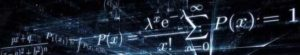# Seminario 4oct2018. Bioestadística e Epidemioloxía. 30 Setembro, 2018 Cinbio Novas 30 setembro 2018O xoves 4 de outubro, 2018 as 10 h., na sala de audiovisuais do Cinbio, módulo 6 (planta 3).

Tito da charla:  “Testing the equality in distribution of a large number of variables for two groups”

Ponente: Marta Cousido, do grupo “SIDOR, Biostatistics and Epidemiology

A conferencia estará en inglés. Resumo:

Nowadays a recurring theme is dealing with high-dimensional data. The main property of high dimensional data is that the data dimension, p, i.e., the number of variables or features (e.g. genes), is large while the sample size is relatively small. In this context we address the problem of testing the null hypothesis that the marginal distributions of p variables are the same for two groups. We propose a test statistic motivated by the simple idea of comparing, for each of the p variables, the empirical characteristic functions computed from the two samples. Our test takes into account that the p variables can be weakly dependent. When the two-sample test accepts the global null hypothesis, the conclusion is that the distribution of each of p variables in the two groups is the same. However, if the test rejects the global null hypothesis additional investigation about which of the p variables have contributed to this significance is required. The individual test statistics forming our global two-sample test can be used to test each of the p null hypotheses separately. More specifically, we define a set of permutation tests based on such individual test statistics. In order to take the multiplicity of tests into account we apply a multiple comparison procedure (MCP) to the set of large-scale homogeneous discrete uniform p-values derived from the application of our permutation tests. In this work we investigate the performance of several MCPs for these type of p-values. Besides, we compare via simulations our permutation test to well-known two sample tests, such as the Kolmogorov-Smirnov test or the classical Student’s t test among others.

Próximos seminarios:

 25 de Outubro QF1 Química Coloidal 8 de Novembro QO1 Química Orgánica 22 de Novembro FB3 Endocrinoloxía